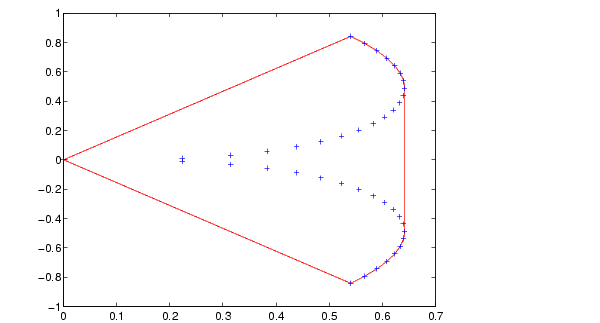MATLAB Function Referenceconvhull

Convex hull

Syntax

• ```K = convhull(x,y)
```K = convhull(x,y,options)
```[K,a] = convhull(...)
```

Description

```K = convhull(x,y) ``` returns indices into the `x` and `y` vectors of the points on the convex hull.

`convhull` uses Qhull.

`K = convhull(x,y,options)` specifies a cell array of strings `options` to be used in Qhull via `convhulln`. The default option is `{'Qt'}`.

If `options` is `[]`, the default options are used. If `options` is `{''}`, no options will be used, not even the default. For more information on Qhull and its options, see http://www.qhull.org.

```[K,a] = convhull(...) ``` also returns the area of the convex hull.

Visualization

Use `plot` to plot the output of `convhull`.

Examples

Example 1.

• ```xx = -1:.05:1; yy = abs(sqrt(xx));
[x,y] = pol2cart(xx,yy);
k = convhull(x,y);
plot(x(k),y(k),'r-',x,y,'b+')```

Example 2. The following example illustrates the `options` input for `convhull`. The following commands

• ```X = [0 0 0 1];
Y = [0 1e-10 0 1];
K = convhull(X,Y)
```

return a warning.

• ```Warning: qhull precision warning:
The initial hull is narrow (cosine of min. angle is
0.9999999999999998).
A coplanar point may lead to a wide facet. Options 'QbB' (scale
to unit box)
or 'Qbb' (scale last coordinate) may remove this warning. Use 'Pp'
to skip
this warning.
```

To suppress this warning, use the option `'Pp'`. The following command passes the option `'Pp'`, along with the default `'Qt'`, to `convhull`.

• ```K = convhull(X,Y,{'Qt','Pp'})

K =

2
1
4
2
```

Algorithm

`convhull` is based on Qhull . For information about Qhull, see http://www.qhull.org/. For copyright information, see http://www.qhull.org/COPYING.txt.

See Also

`convhulln`, `delaunay`, `plot`, `polyarea`, `voronoi`

Reference

  Barber, C. B., D.P. Dobkin, and H.T. Huhdanpaa, "The Quickhull Algorithm for Convex Hulls," ACM Transactions on Mathematical Software, Vol. 22, No. 4, Dec. 1996, p. 469-483. Available in PDF format at http://www.acm.org/pubs/ citations/journals/toms/1996-22-4/p469-barber/.

  National Science and Technology Research Center for Computation and Visualization of Geometric Structures (The Geometry Center), University of Minnesota. 1993.

© 1994-2005 The MathWorks, Inc.